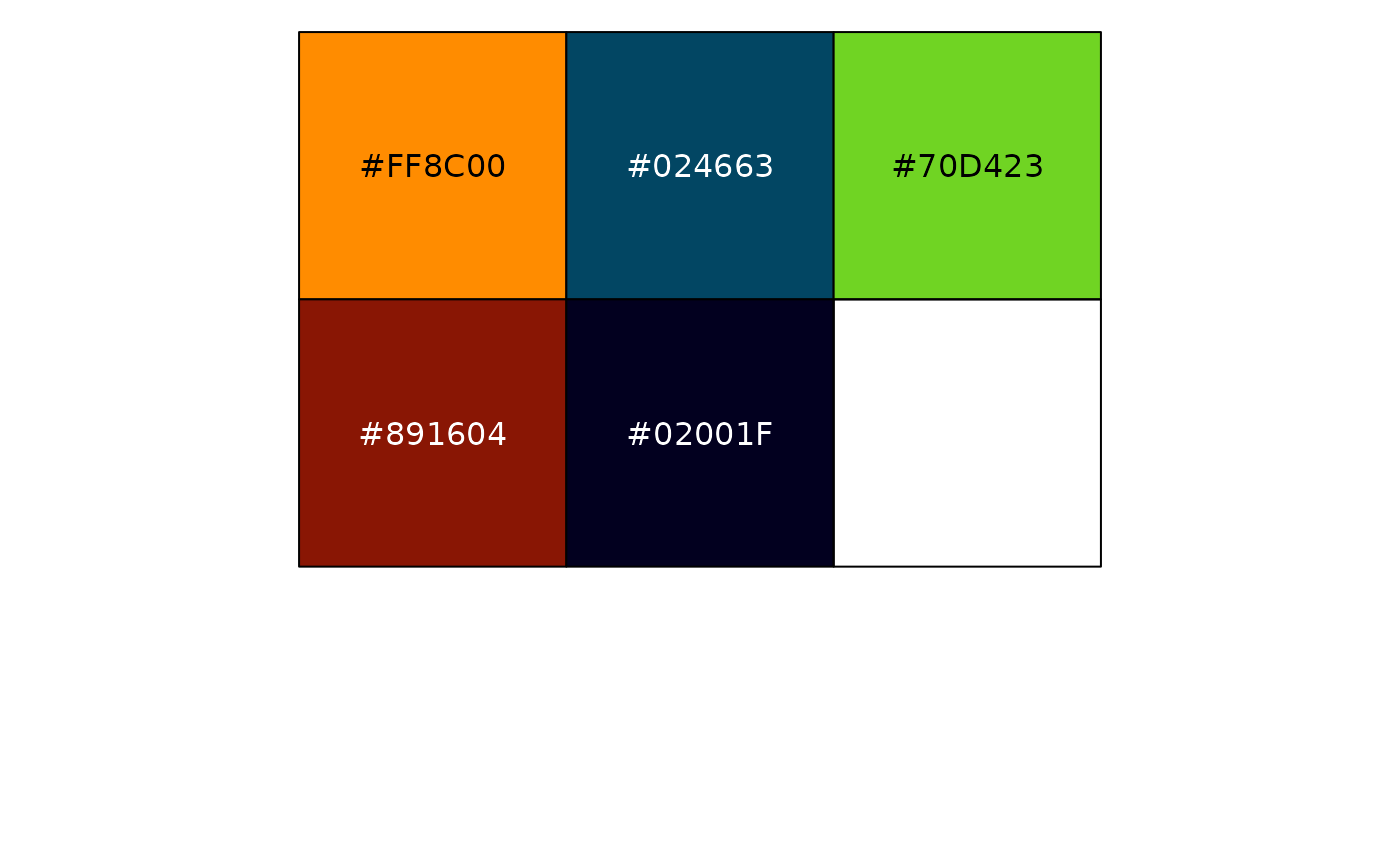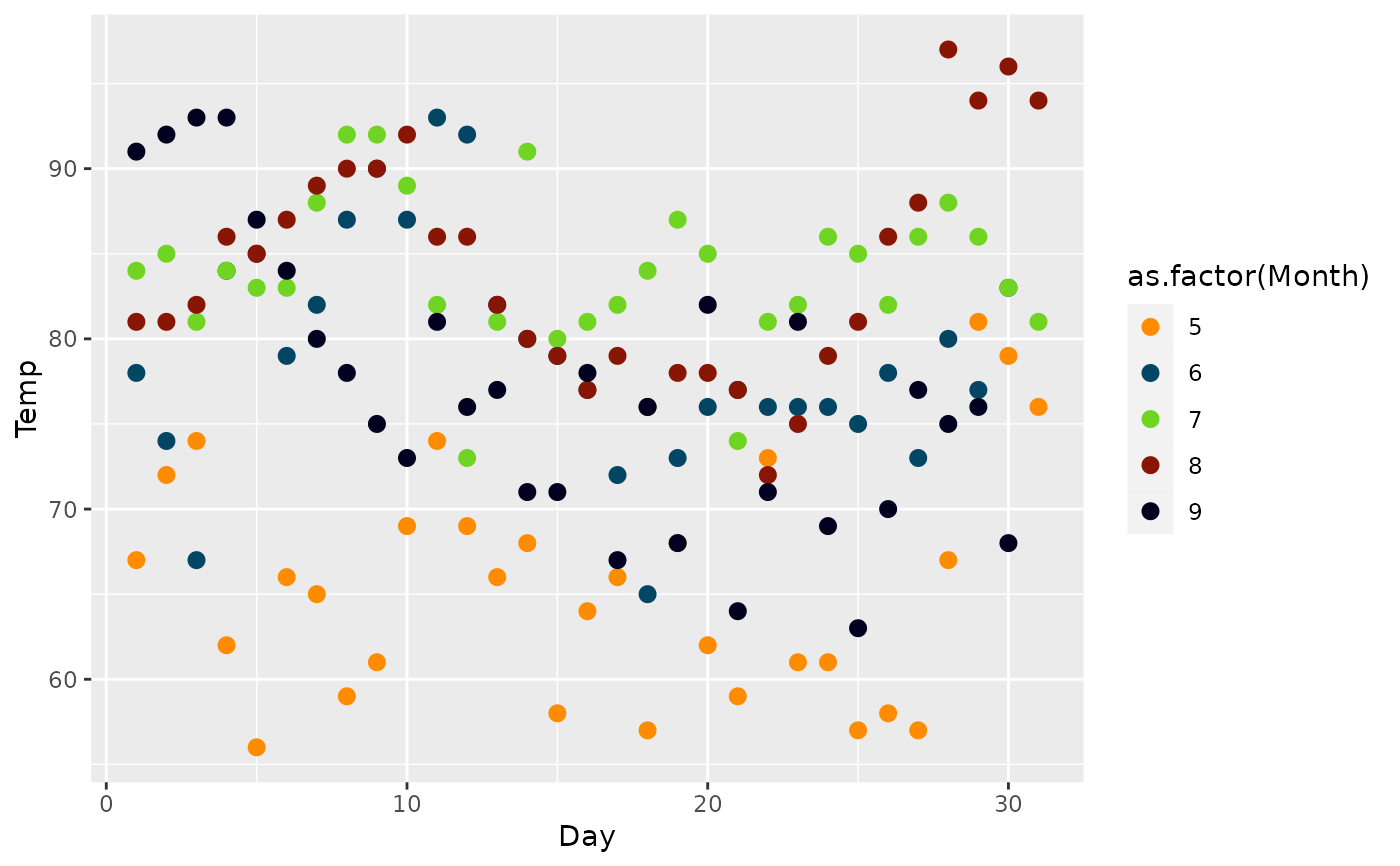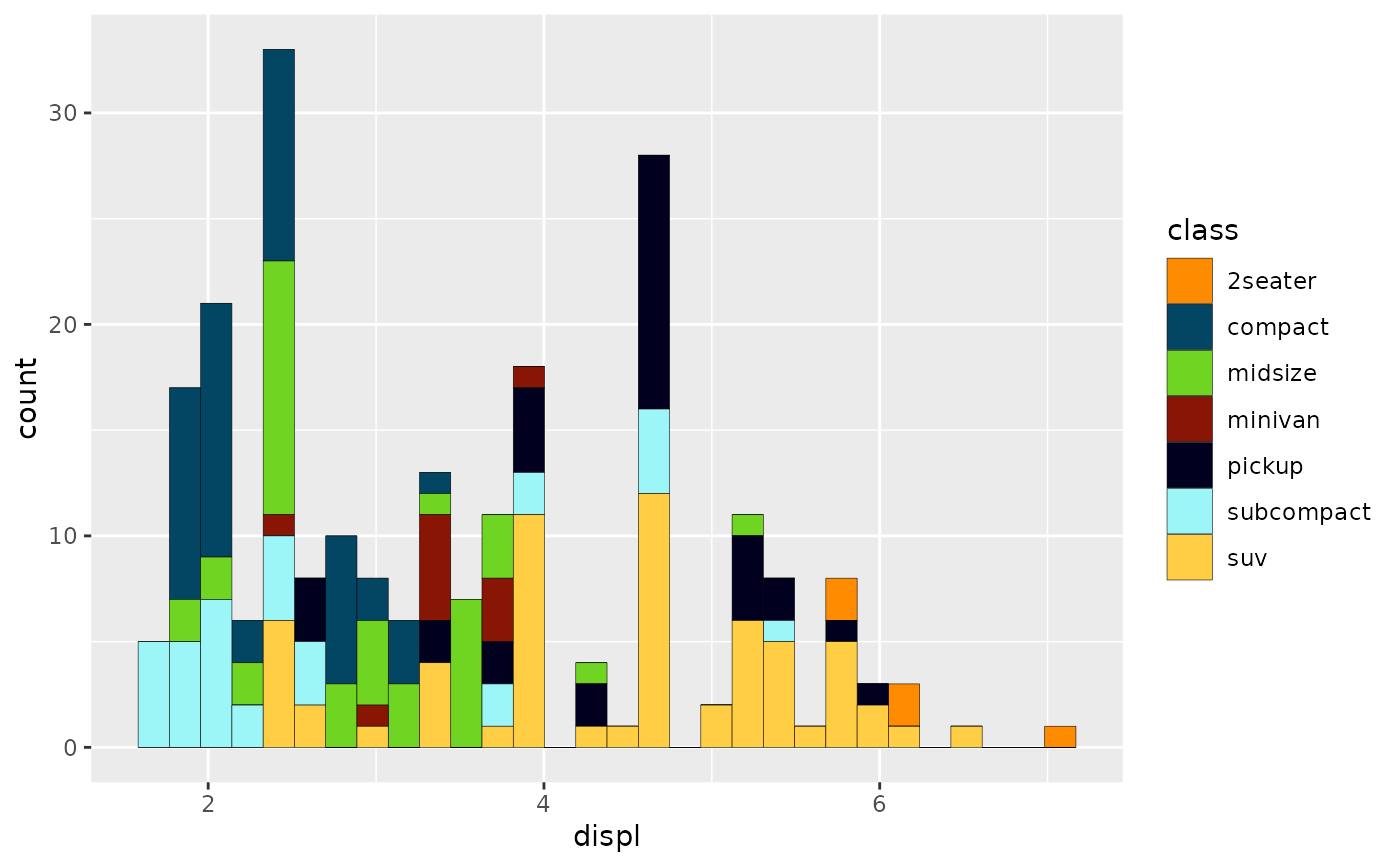Kim Possible palette

kimPossible_pal(n, type = c("discrete", "continuous"), reverse = FALSE)

scale_color_kimPossible(n, type = "discrete", reverse = FALSE, ...)

scale_colour_kimPossible(n, type = "discrete", reverse = FALSE, ...)

scale_fill_kimPossible(n, type = "discrete", reverse = FALSE, ...)

## Arguments

n number of colors discrete or continuous reverse order, Default: FALSE Arguments passed on to ggplot2::discrete_scale aestheticsThe names of the aesthetics that this scale works with. scale_nameThe name of the scale that should be used for error messages associated with this scale. paletteA palette function that when called with a single integer argument (the number of levels in the scale) returns the values that they should take (e.g., scales::hue_pal()). nameThe name of the scale. Used as the axis or legend title. If waiver(), the default, the name of the scale is taken from the first mapping used for that aesthetic. If NULL, the legend title will be omitted. breaksOne of: NULL for no breaks waiver() for the default breaks (the scale limits) A character vector of breaks A function that takes the limits as input and returns breaks as output labelsOne of: NULL for no labels waiver() for the default labels computed by the transformation object A character vector giving labels (must be same length as breaks) A function that takes the breaks as input and returns labels as output limitsA character vector that defines possible values of the scale and their order. expandFor position scales, a vector of range expansion constants used to add some padding around the data to ensure that they are placed some distance away from the axes. Use the convenience function expansion() to generate the values for the expand argument. The defaults are to expand the scale by 5% on each side for continuous variables, and by 0.6 units on each side for discrete variables. na.translateUnlike continuous scales, discrete scales can easily show missing values, and do so by default. If you want to remove missing values from a discrete scale, specify na.translate = FALSE. na.valueIf na.translate = TRUE, what value aesthetic value should missing be displayed as? Does not apply to position scales where NA is always placed at the far right. dropShould unused factor levels be omitted from the scale? The default, TRUE, uses the levels that appear in the data; FALSE uses all the levels in the factor. guideA function used to create a guide or its name. See guides() for more information. positionFor position scales, The position of the axis. left or right for y axes, top or bottom for x axes. superThe super class to use for the constructed scale

## Examples

library(scales)
show_col(kimPossible_pal()(5))library(ggplot2)
ggplot(airquality, aes(x = Day, y = Temp,
group = as.factor(Month), color = as.factor(Month))) +
geom_point(size = 2.5) +
scale_color_kimPossible()ggplot(airquality, aes(x = Day, y = Temp,
group = as.factor(Month), color = as.factor(Month))) +
geom_point(size = 2.5) +
scale_colour_kimPossible()ggplot(mpg, aes(displ)) +
geom_histogram(aes(fill = class), col = "black", size = 0.1) +
scale_fill_kimPossible()#> stat_bin() using bins = 30. Pick better value with binwidth.